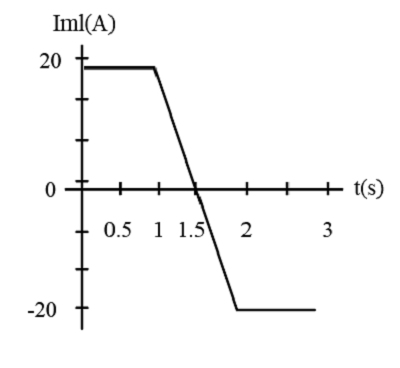# A 4.0-cm-diameter loop with resistance 0.14 \Omega around a 2.0-cm-diameter solenoid. The...

## Question:

A 4.0-cm-diameter loop with resistance 0.14 {eq}\Omega {/eq} around a 2.0-cm-diameter solenoid. The solenoid is 14 cm long, has 100 turns, and carries the current. A positive current is cw when seen from the left.

Find the current in the loop at t = 0.5s.

Find the current in the loop at t = 1.5s.

Find the current in the loop at t = 2.5s.

## Current:

In electrical engineering, the current is defined as the ratio of electric charge flowing in a circuit in a unit time. The current flowing in a circuit is measured in terms of an ampere in the standard international system.

Current:

In electrical engineering, the current is defined as the ratio of electric charge flowing in a circuit in a unit time. The current flowing in a circuit is measured in terms of ampere in the standard international system.

Given Data

• The diameter of the loop is {eq}d' = 4\;{\rm{cm}} = 4 \times {10^{ - 2}}\;{\rm{m}} {/eq}.
• The diameter of the solenoid is {eq}d = 2\;{\rm{cm}} = 2 \times {10^{ - 2}}\;{\rm{m}} {/eq}.
• The length of the solenoid is {eq}{l} = 14\;{\rm{cm}} = 14 \times {10^{ - 2}}\;{\rm{m}} {/eq}.
• The turns of solenoid is {eq}N = 100 {/eq}.
• The resistance in the loop is {eq}R = 0.14\;\Omega {/eq}
• The time of the loop is {eq}t = 0.5\;{\rm{s}} {/eq}.The expression of the magnetic field is given by.

{eq}B = {\mu _o}\left( {\dfrac{N}{l}} \right)I {/eq}

The expression of the magnetic flux is given by.

{eq}\phi = BA {/eq}

The area is calculated as.

{eq}A = \pi {r^2} {/eq}

Substitute the value in the above expression.

{eq}\phi = {\mu _o}\left( {\dfrac{N}{l}} \right)I\left( {\pi {r^2}} \right) {/eq}

The expression of induced current is calculated as.

{eq}{I_a} = \dfrac{1}{R}\left( {\dfrac{{d\phi }}{{dt}}} \right) {/eq}

Substitute the value in the above expression.

{eq}\begin{align*} {I_a}& = \dfrac{1}{R}\left( {\dfrac{d}{{dt}}{\mu _o}\left( {\dfrac{N}{l}} \right)I\left( {\pi {r^2}} \right)} \right)\\ {I_a} &= \dfrac{{{\mu _o}N\pi {r^2}}}{{Rl}}\dfrac{{dI}}{{dt}}.....(1) \end{align*} {/eq}

When the change in current {eq}\dfrac{{dI}}{{dt}} {/eq} at {eq}t = 0.5\;{\rm{s}} {/eq} is zero.

Substitute the value in the equation (1).

{eq}\begin{align*} {I_a}_{_1} &= \dfrac{{{\mu _o}N\pi {r^2}}}{{Rl}} \times 0\\ {I_a}_{_1} &= 0 \end{align*} {/eq}

Thus, the current is in the loop is zero.

When the time is {eq}t = 1.5\;{\rm{s}} {/eq} then the change in current is {eq}\dfrac{{dI}}{{dt}} = 40 {/eq}.

Substitute the value in the equation (1).

{eq}\begin{align*} {I_a}_{_2} &= \dfrac{{\left( {4\pi \times {{10}^{ - 7}}} \right)\left( {100} \right)\pi {{\left( {1 \times {{10}^{ - 2}}\;{{\rm{m}}^2}} \right)}^2}}}{{\left( {0 \cdot 14\;\Omega } \right)\left( {14 \times {{10}^{ - 2}}\;{\rm{m}}} \right)}} \times \left( {40\;{\rm{A}}} \right)\\ {I_a}_{_2} &= 8.056 \times {10^{ - 5}}\;{\rm{A}} \end{align*} {/eq}

Thus, the current is in the loop is {eq}8.056 \times {10^{ - 5}}\;{\rm{A}} {/eq}.

When the change in current {eq}\dfrac{{dI}}{{dt}} {/eq} at {eq}t = 2.5\;{\rm{s}} {/eq} is zero.

Substitute the value in the equation (1).

{eq}\begin{align*} {I_a}_{_3} &= \dfrac{{{\mu _o}N\pi {r^2}}}{{Rl}} \times 0\\ {I_a}_{_3}& = 0 \end{align*} {/eq}

Thus, the current is in the loop is zero.